## RS Aggarwal Solutions Class 10 Chapter 17 Perimeter and Areas of Plane Figures Ex 17a

These Solutions are part of RS Aggarwal Solutions Class 10. Here we have given RS Aggarwal Solutions Class 10 Chapter 17 Perimeter and Areas of Plane Figures Ex 17a.

Other Exercises

Question 1.
Solution:Question 2.
Solution:
Let a = 42 cm, b = 34 cm and c = 20 cm(ii)Let base = 42 cm and corresponding height = h cm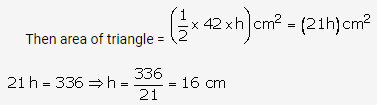Hence, the height corresponding to the longest side = 16 cm

Question 3.
Solution:
Let a = 18 cm, b = 24 cm, c = 30 cm
Then,2s = (18 + 24 + 30) cm = 72 cm
s = 36 cm
(s a) = 18cm, (s b) = 12 cm and (s c) = 6 cm(ii)Let base = 18 cm and altitude = x cm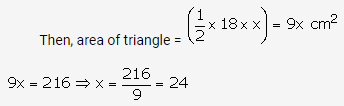Hence, altitude corresponding to the smallest side = 24 cm

Question 4.
Solution:
On dividing 150 m in the ratio 5 : 12 : 13, we getQuestion 5.
Solution:
On dividing 540 m in ratio 25 : 17 : 12, we get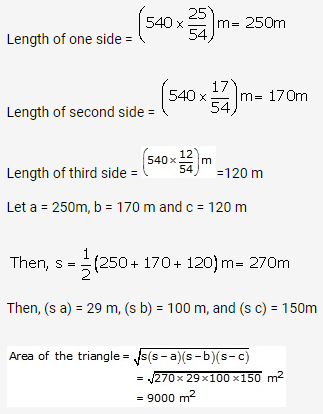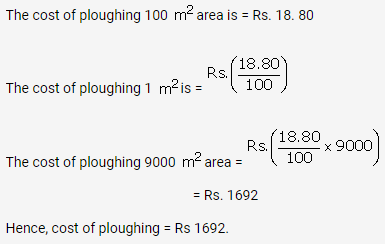Question 6.
Solution:
Let the length of one side be x cm
Then the length of other side = {40 (17 + x)} cm = (23 – x) cm
Hypotenuse = 17 cm
Applying Pythagoras theorem, we get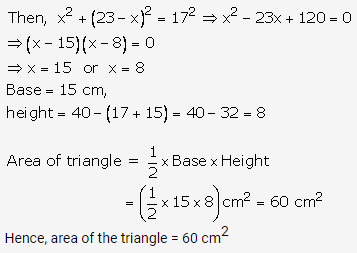Question 7.
Solution:
Let the sides containing the right – angle be x cm and (x – 7) cm∴ perimeter of triangle (15 + 8 + 17) cm = 40 cm

Question 8.
Solution:
Let the sides containing the right angle be x and (x 2) cmTherefore, perimeter of the triangle = 8 + 6 + 10 = 24 cm

Question 9.
Solution:
Side of an equilateral triangle = a = 10 cm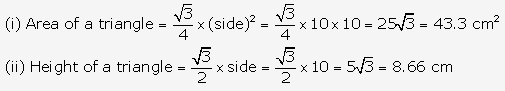Question 10.
Solution:
Let each side of the equilateral triangle be a cm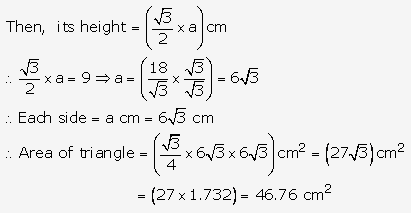Question 11.
Solution:
Let each side of the equilateral triangle be a cm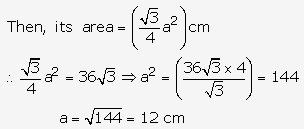Perimeter of equilateral triangle = 3a = (3 12) cm = 36 cm

Question 12.
Solution:
Let each side of the equilateral triangle be a cm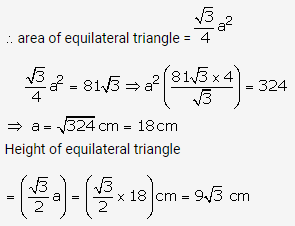Question 13.
Solution:
Base of right angled triangle = 48 cm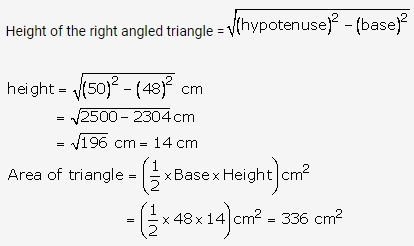Question 14.
Solution:
Let the hypotenuse of right – angle triangle = 6.5 m
Base = 6 cmHence, perpendicular = 2.5 cm and area of the triangle =7.5 cm2

Question 15.
Solution:
The circumcentre of a right – triangle is the midpoint of the hypotenuse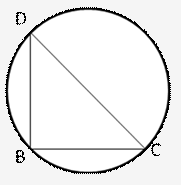Hypotenuse = 2 × (radius of circumcircle)
= (2 × 8) cm = 16 cm
Base = 16 cm, height = 6 cm
Area of right angled triangle=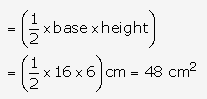Hence, area of the triangle= 48 cm2

Question 16.
Solution:
Let each equal side be a cm in length.
Then,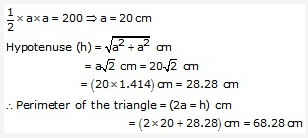Hence, hypotenuse = 28.28 cm and perimeter = 68.28 cm

Question 17.
Solution:
Let each equal side be a cm and base = 80 cm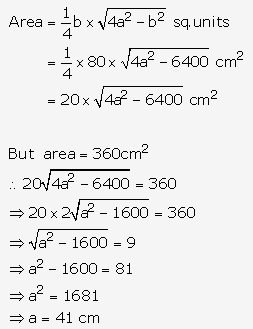perimeter of triangle = (2a + b) cm
= (2 41 + 80) cm
= (82 + 80) cm = 162 cm
Hence, perimeter of the triangle = 162 cm

Question 18.
Solution:
Let the height be h cm, then a= (h + 2) cm and b = 12 cmHence, area of the triangle = 48 cm2.

Question 19.
Solution:
Let ∆ABC is a isosceles triangle. Let AC, BC be the equal sides
Then AC = BC = 10cm. Let AB be the base of ∆ABC right angle at C.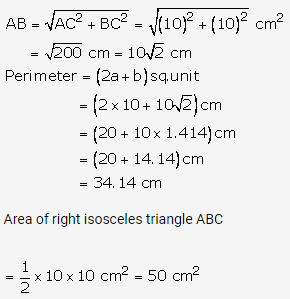Hence, area = 50 cm2 and perimeter = 34.14 cm

Question 20.
Solution: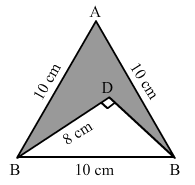Area of shaded region = Area of ABC – Area of DBC
First we find area of ABCArea of shaded region = Area of ∆ABC – Area of ∆DBC
= (43.30 – 24) cm2 = 19. 30 cm2
Area of shaded region = 19.3 cm2

Hope given RS Aggarwal Solutions Class 10 Chapter 17 Perimeter and Areas of Plane Figures Ex 17a are helpful to complete your math homework.

If you have any doubts, please comment below. Learn Insta try to provide online math tutoring for you.